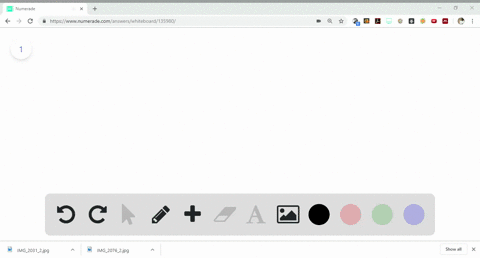Sign up for our free STEM online summer camps starting June 1st!View Summer Courses### Write balanced chemical equations to correspond t…

13:46University of New Mexico

Need more help? Fill out this quick form to get professional live tutoring.

Get live tutoring
Problem 15

Write balanced chemical equations corresponding to each of the following descriptions: (a) Solid calcium carbide, $\mathrm{CaC}_{2}$ , reacts with water to form an aqueous solution of calcium hydroxide and acetylene gas, $\mathrm{C}_{2} \mathrm{H}_{2}$ . (b) When solid potassium chlorate is heated, it decomposes to form solid potassium chloride and oxygen gas. (c) Solid zinc metal reacts with sulfuric acid to form hydrogen gas and an aqueous solution of zinc sulfate. (d) When liquid phosphorus trichloride is added to water, it reacts to form aqueous phosphorous acid, $\mathrm{H}_{3} \mathrm{PO}_{3}(a q)$, and aqueous hydrochloric acid. (e) When hydrogen sulfide gas is passed over solid hot iron(III) hydroxide, the resulting reaction produces solid iron(II) sulfide and gaseous water.

a) $\mathrm{CaC}_{2}+2 \mathrm{H}_{2} \mathrm{O} \rightarrow \mathrm{Ca}(\mathrm{OH})_{2}+\mathrm{C}_{2} \mathrm{H}_{2}$
b) See explanation for result.
c) $Z n(s)+H_{2} S O_{4}(a q) \rightarrow H_{2}(g)+Z n S O_{4}(a q)$
d) $P C l_{3}(l)+H_{2} O(l) \rightarrow H_{3} P O_{3}(a q)+H C l(a q)$
e) $H_{2} S(g)+F e(O H)_{3}(s) \rightarrow F e_{2} S_{3}(s)+H_{2} O(g)$

## Discussion

You must be signed in to discuss.

## Video Transcript

the balance equation are represented, as followed for auction e this two. It's too low giving this see you. Which wards? Group less C two. It's too for option B, two K, C E o or three representing two key seal last three or two for auctions. Steam it is the den plus which two? A school full giving this it's too plus, is that then it's cool. Four. Auction being be seal three less three edge, too. Next three people three plus three EJ seal for the last option. It ISS three h two s just two f e Which well, pew giving this Effie too s three. That's six h 20